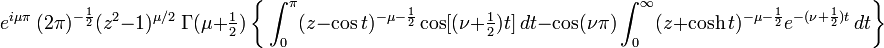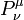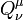# User:Tohline/Math/EQ Toroidal04

 Failed to parse (unknown function\vu): ~(\nu - \mu + 1)P^\mu_{\vu + 1}$~=$$~ e^{i \mu \pi} ~ (2\pi)^{-\frac{1}{2}} (z^2-1)^{\mu/2} ~\Gamma(\mu + \tfrac{1}{2})~\biggl\{ \int_0^\pi (z - \cos t)^{-\mu - \frac{1}{2}} \cos[(\nu + \tfrac{1}{2})t] ~dt -\cos(\nu\pi) \int_0^\infty (z + \cosh t)^{-\mu - \frac{1}{2}} e^{-(\nu + \frac{1}{2})t} ~dt \biggr\}$ Abramowitz & Stegun (1995), p. 334, eq. (8.5.3)
 NOTE: Both$~P_\nu^\mu$ and$~Q_\nu^\mu$ satisfy this same recurrence relation.